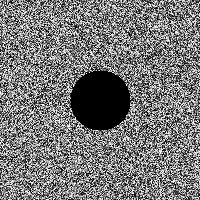# ds_grid_drawThis script will draw the contents of a grid data structure which is populated by numerical data. It performs a normalization process so that the entire range of values within the grid are drawn from black to white.

While the script is very simple and lacks options, it can be especially useful for development and debugging purposes.

ds_grid_draw(grid, x, y)
Draws the data of a given grid at a screen location.
COPY/// @func   ds_grid_draw(grid, x, y)
///
/// @desc   Draws the data of a given grid at a screen location.
///
/// @param  {grid}      grid        grid data structure
/// @param  {real}      x           screen position x-coordinate
/// @param  {real}      y           screen position y-coordinate
///

function ds_grid_draw(grid, x, y)
{
var w = ds_grid_width(grid);
var h = ds_grid_height(grid);

var M = ds_grid_get_max(grid, 0, 0, w-1, h-1);
var m = ds_grid_get_min(grid, 0, 0, w-1, h-1);
if (M == m) var f = 0 else var f = 1 / (M - m);

for (var i=0; i<w; i++)
{
for (var j=0; j<h; j++)
{
var value = f * (ds_grid_get(grid, i, j) - m);
draw_point_color(x+i, y+j, make_color_hsv(0, 0, clamp(255*value, 0, 255)));
}
}
}


Contributors: xot

GitHub: View · Commits · Blame · Raw